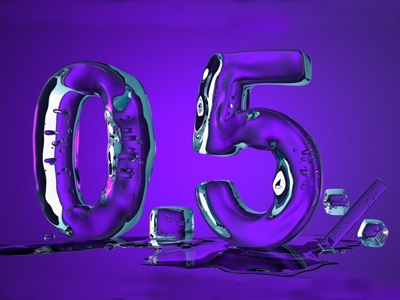0.5% is a very small fraction, worth the same as 1200

# Percentages 2 (Medium)

This is the second of our medium level Eleven Plus maths quizzes on Percentages. If you have already played the first, then you will know that percentages are a way of representing parts of numbers, just like fractions or decimals. The difference is that, in percentages, the parts of numbers are shown as parts of one hundred.

To help you visualise, here are some fractions represented as decimals and as percentages:

• 12 = 0.5 = 50%
• 14 = 0.25 = 25%
• 13 = 0.333 = 33.3%

As you can see, percentages are very similar to decimals. The only difference is where the decimal point is placed.

Now it’s time to work some percentages out for yourself. When you can get all the answers right, why not move on to the next quiz? You’ll soon be a whizz with percentages.

1.
What is 17% of 120?
19
20.4
21.8
23.2
17% of 120 = (17 ÷ 100) × 120 = (17 × 120) ÷ 100 = 20.4. You might have had to use your calculator on this question
2.
What is 14% of 400?
56
70
84
98
14% of 400 = (14 ÷ 100) × 400 = (400 × 14) ÷ 100 = 56
3.
What is 17% of 93?
15
15.81
16
16.81
17% of 93 = (17 ÷ 100) × 93 = (17 × 93) ÷ 100 = 15.81
4.
What is 75% of 75?
56.25
1
50
57.5
75% of 75 = (75 ÷ 100) × 75 = (75 × 75) ÷ 100 = 56.25
5.
There are 30 children in a class. If 30% of them have a pet dog, how many children do not have a pet dog?
9
10
20
21
100% - 30% = 70%.
70% of 30 = (70 ÷ 100) x 30 = (70 x 30) ÷ 100 = 21
6.
Last year, Susan was 138 cm tall. Since then her height has increased by 5%. How tall is Susan now?
6.9 cm
143 cm
144.9 cm
145.8 cm
5% of 138 cm = (5 ÷ 100) × 138 = (5 × 138) ÷ 100 = 6.9 cm. This is the amount Susan has grown by.
To work out Susan’s height now, simply add 6.9 to 138:
138 cm + 6.9 cm = 144.9 cm
7.
Mum’s car cost her £28,000 when she bought it three years ago. Since then, its value has decreased by 24%. How much is Mum’s car worth now?
£21,280
£6,720
£34,720
£18,280
24% of £28,000 = (24 ÷ 100) × 28,000 = (24 × 28,000) ÷ 100 = £6,720. This is the amount the car’s value has decreased by.
To work out how much the car is now worth, simply subtract 6,720 from 28,000:
28,000 – 6,720 = 21,280. Don’t forget to convert your answer back into pounds: £21,280
8.
What is 60% of 25?
18
15
12
21
60% of 25 = (60 ÷ 100) × 25 = (60 × 25) ÷ 100 = 15. DON'T forget: when you divide by 100, move the digits two places to the right
9.
What is 10% of 10% of 100?
10
5
2
1
10% of 10% = 1%
Of course, you know that 1% of 100 = 1
10.
Sandra’s pocket money is to go up by 15%. At the moment she gets £7.50 per week. What will she get in future?
£7.65 per week
£1.13 per week
£8.63 per week
£8.75 per week
15% of £7.50 = (15 ÷ 100) × 7.5 = (15 × 7.5) ÷ 100 = 1.125
Next, you add this amount to Sandra’s pocket money: 7.5 + 1.125 = 8.625.
Don’t forget to convert your answer back into pounds and pence. In this case, you will have to round your answer up to £8.63
Author:  Frank Evans